# Get minimum values in rows or columns with their index position in Pandas-Dataframe

Let’s discuss how to find minimum values in rows & columns of a Dataframe and also their index position.

a) Find the minimum value among rows and columns :

Dataframe.min() : This function returns the minimum of the values in the given object. If the input is a series, the method will return a scalar which will be the minimum of the values in the series. If the input is a dataframe, then the method will return a series with a minimum of values over the specified axis in the dataframe. By default, the axis is the index axis.

1) Get minimum values of every column :
Use min() function to find the minimum value over the index axis.

Code :

 # import pandas library  import pandas as pd     # list of Tuples  data = [            (20, 16, 23),            (30, None, 11),            (40, 34, 11),            (50, 35, None),            (60, 40, 13)          ]     # creating a DataFrame object  df = pd.DataFrame(data, index = ['a', 'b', 'c', 'd', 'e'],                       columns = ['x', 'y', 'z'])     # getting a series object containing   # minimum value from each column  # of given dataframe  minvalue_series = df.min()     minvalue_series

Output: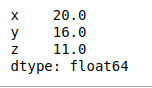2) Get minimum values of every row :
Use min() function on a dataframe with ‘axis = 1’ attribute to find the minimum value over the row axis.

Code :

 # import pandas library  import pandas as pd     # list of Tuples  data = [            (20, 16, 23),            (30, None, 11),            (40, 34, 11),            (50, 35, None),            (60, 40, 13)          ]     # creating a DataFrame object  df = pd.DataFrame(data, index = ['a', 'b', 'c', 'd', 'e'],                       columns = ['x', 'y', 'z'])     # getting a series object containing   # minimum value from each row  # of given dataframe  minvalue_series = df.min(axis = 1)     minvalue_series

Output: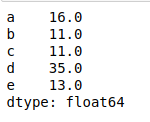3) Get minimum values of every column without skipping None Value :
Use min() function on a dataframe which has Na value with ‘skipna = False’ attribute to find the minimum value over the column axis.

Code :

 # import pandas library  import pandas as pd     # list of Tuples  data = [            (20, 16, 23),            (30, None, 11),            (40, 34, 11),            (50, 35, None),            (60, 40, 13)          ]     # creating a DataFrame object  df = pd.DataFrame(data, index = ['a', 'b', 'c', 'd', 'e'],                       columns = ['x', 'y', 'z'])     # getting a series object containing   # minimum value from each column  # of given dataframe without  # skipping None value  minvalue_series = df.min(skipna = False)     minvalue_series

Output: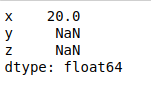4) Get minimum value of a single column :
Use min() function on a series to find the minimum value in the series.

Code :

 # import pandas library  import pandas as pd     # list of Tuples  data = [            (20, 16, 23),            (30, None, 11),            (40, 34, 11),            (50, 35, None),            (60, 40, 13)          ]     # creating a DataFrame object  df = pd.DataFrame(data, index = ['a', 'b', 'c', 'd', 'e'],                       columns = ['x', 'y', 'z'])     # getting a minimum value  # from column 'x'  minvalue = df['x'].min()     minvalue

Output:

20

b) Get row index label or position of minimum values among rows and columns :

Dataframe.idxmin() : This function returns index of first occurrence of minimum over requested axis. While finding the index of the minimum value across any index, all NA/null values are excluded.

1) Get row index label of minimum value in every column :
Use idxmin() function to find the index/label of the minimum value along the index axis.

Code :

 # import pandas library  import pandas as pd     # list of Tuples  data = [            (20, 16, 23),            (30, None, 11),            (40, 34, 11),            (50, 35, None),            (60, 40, 13)          ]     # creating a DataFrame object  df = pd.DataFrame(data, index = ['a', 'b', 'c', 'd', 'e'],                       columns = ['x', 'y', 'z'])     # get the index position\label of  # minimum values in every column  minvalueIndexLabel = df.idxmin()     minvalueIndexLabel

Output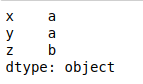2) Get Column names of minimum value in every row :
Use idxmin() function with ‘axis = 1’ attribute to find the index/label of the minimum value along the column axis.

Code :

 # import pandas library  import pandas as pd     # list of Tuples  data = [            (20, 16, 23),            (30, None, 11),            (40, 34, 11),            (50, 35, None),            (60, 40, 13)          ]     # creating a DataFrame object  df = pd.DataFrame(data, index = ['a', 'b', 'c', 'd', 'e'],                       columns = ['x', 'y', 'z'])     # get the index position\label of  # minimum values in every row  minvalueIndexLabel = df.idxmin(axis = 1)     minvalueIndexLabel

Output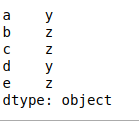Whether you're preparing for your first job interview or aiming to upskill in this ever-evolving tech landscape, GeeksforGeeks Courses are your key to success. We provide top-quality content at affordable prices, all geared towards accelerating your growth in a time-bound manner. Join the millions we've already empowered, and we're here to do the same for you. Don't miss out - check it out now!

Previous
Next